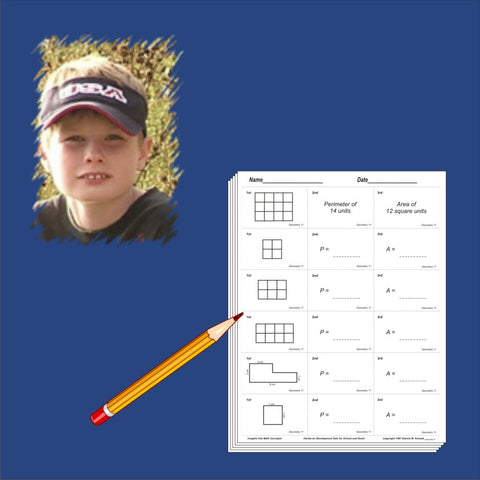## Discounted Fourth Grade Blackline Set

• 39000

• Third grade blacklines for reinforcement
• Photocopy for written work. Also includes answer sheets.
• Numerous exercise sets
Thousands (green), Problem Solving C, Mixed Practice C, Geometry Introduction, Money C, Time C, Fraction Match, Roman Numerals, Problem Solving D, Mixed Practice D, Fraction Concepts, Decimal Introduction, Estimation, Advanced Numeration, Fraction Number Lines, Order Fractions, Fraction Operations, More Fraction Operations, Number Theory, Decimal Line & Labels, Order Decimals, Problem Solving E, Mixed Practice E